Learn data science and machine learning by building real-world projects on Jovian

## Classifying 10 Famous Personality Image Dataset using Residual Network in PyTorch

A.K.A. Training an image classifier from scratch to over 90% accuracy in around 1 minute on a single GPU

In this project, we'll use the following techniques to train a state-of-the-art model in around 1 minute to achieve over 90% accuracy in classifying images from the 10 Famous personality Image Dataset,

• Data normalization
• Data augmentation
• Residual connections
• Batch normalization
• Learning rate scheduling
• Weight Decay
In :
``project_name='10-famous-personality-classification'``
In :
``````import torch
import torchvision
import numpy as np
import matplotlib
import matplotlib.pyplot as plt
matplotlib.rcParams['figure.facecolor'] = '#ffffff'

%matplotlib inline
``````

### Fetching Kaggle Datasets into Google Colab

#### 1. Get you Kaggle API Token

• Go to Your Account and click on Create New API Token.

• Create a folder named Kaggle where we will be storing our Kaggle datasets

Below cell will mount the Google Drive to Google Colab. The steps are,

1. Run the script,
• `from google.colab import drive \ drive.mount('/content/gdrive')`
2. Copy the authentication code
3. Paste the code into the input shell
In :
``````from google.colab import drive
drive.mount('/content/gdrive')``````
```Mounted at /content/gdrive ```

#### 4. Provide the config path to kaggle.json

Below cell will set the kaggle configuration path to kaggle.json

In :
``````import os
os.environ['KAGGLE_CONFIG_DIR'] = "/content/gdrive/My Drive/Kaggle"``````

#### 5. Changing present working directory

Below cell will set the present working directory to,
/content/gdrive/My Drive/Kaggle

In :
``%cd /content/gdrive/My Drive/Kaggle``
```/content/gdrive/My Drive/Kaggle ```

1. Go to kaggle and copy the API Command to download the dataset
2. Your API Command will look like `kaggle datasets download -d <username>/<datasets>`

I have used the Famous Personalities Image Dataset from Kaggle

In :
``````if os.path.exists('Famous Personality'):
os.system("rm -r 'Famous Personality'")
```Downloading famous-personalities-image-dataset.zip to Famous Personality 97% 122M/126M [00:06<00:00, 23.9MB/s] 100% 126M/126M [00:06<00:00, 19.5MB/s] ```

### Description of the Dataset

This dataset folder contains images of 10 famous personalities which are listed below.

1. Anushka Sharma (Actress-India)
2. Barack Obama (Former USA President)
3. Bill Gates (Philanthropist)
5. Indira Nooyi (CEO-Pepsico)
6. Melinda Gates (Philanthropist)
7. Narendra Modi (Prime Minister of India)
9. Vikas Khanna (Celebrity Chef)
10. Virat Kohli (Cricketer)

The cropped folder contains cropped faces images of the personalities to directly train into the model.

In :
``root="Famous Personality/"``

List the extracted files and folder

In :
``os.listdir(root)``
Out:
``['Dataset', 'cropped', 'haarcascade_frontalface_default.xml']``
In :
``os.listdir(root+'Dataset/Dataset')``
Out:
``````['Anushka_Sharma',
'Barack_Obama',
'Bill_Gates',
'Dalai_Lama',
'Indira_Nooyi',
'Melinda_Gates',
'Narendra_Modi',
'Sundar_Pichai',
'Vikas_Khanna',
'Virat_Kohli']``````
In :
``os.listdir(root+'cropped/cropped')``
Out:
``````['Anushka_Sharma',
'Barack_Obama',
'Bill_Gates',
'Dalai_Lama',
'Indira_Nooyi',
'Melinda_Gates',
'Narendra_Modi',
'Sundar_Pichai',
'Vikas_Khanna',
'Virat_Kohli']``````

### Data Preprocessing - Generating cropped face images

Data preprocessing involves getting the cropped face image from the raw image, inorder to train model using only the cropped face image, if face is recognized from the image.Though the dataset has already contained with the cropped face images in /cropped/cropped location, we will be generating fresh cropped face images with this step. Skip this section if you don't want to deep dive into Data preprocessing task.

#### Using Opencv haarcascade to recognize face in an image

Object Detection using Haar feature-based cascade classifiers is an effective object detection method proposed by Paul Viola and Michael Jones in their paper, `Rapid Object Detection using a Boosted Cascade of Simple Features` in 2001. It is a machine learning based approach where a cascade function is trained from a lot of positive and negative images. It is then used to detect objects in other images.

Reference:

In :
``````import cv2

The following function returns `face image` in gray scale, if only one face is recognized otherwise return `None`

In :
``````def get_face(image_path):
face = None
if img is not None:
if len(faces)==1:
x,y,w,h=faces
face = img[y:y+h, x:x+w]
return face``````

Lets see a picture from the raw dataset

In :
``````path=root+'Dataset/Dataset/Bill_Gates/bigates_067.jpg'
``````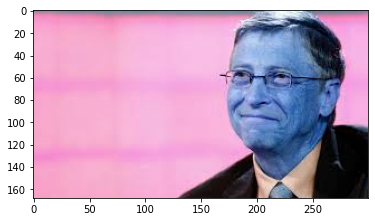Color mismatch is due to `OpenCV` library read images in `BGR` format while `pyplot` library read images in `RGB` format.

Lets use `get_face` helper function to extract face from the image

In :
``````crop=get_face(path)
if crop is not None:plt.imshow(crop)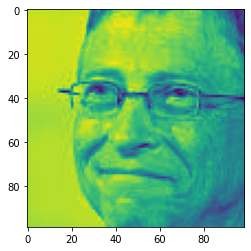#### Generating cropped face images

Run the below cell, if you we want to generate cropped face images and store them in the `cropped` folder. This process will take approximately 2 to 3 minutes.

In :
``````%%time
src='Dataset/Dataset/'
des='cropped/'
if os.path.exists(root+des):
os.system(f"rm -r '{root+des}'")
os.mkdir(root+des)
for folder in os.listdir(root+src):
os.mkdir(root+des+folder)
c=0
for f in os.listdir(root+src+folder):
src_path=os.path.join(root,src,folder,f)
crop=get_face(src_path)
if crop is not None:
c+=1
des_path=os.path.join(root,des,folder,f"{c:03d}.png")
cv2.imwrite(des_path,crop)
print(f"Folder: {folder}, {c} images cropped")``````
```Folder: Anushka_Sharma, 198 images cropped Folder: Barack_Obama, 314 images cropped Folder: Bill_Gates, 262 images cropped Folder: Dalai_Lama, 240 images cropped Folder: Indira_Nooyi, 226 images cropped Folder: Melinda_Gates, 334 images cropped Folder: Narendra_Modi, 212 images cropped Folder: Sundar_Pichai, 208 images cropped Folder: Vikas_Khanna, 239 images cropped Folder: Virat_Kohli, 278 images cropped CPU times: user 3min 52s, sys: 2.8 s, total: 3min 55s Wall time: 2min 51s ```

Number of files in each Folder of `cropped` folder

In :
``````for folder in os.listdir(root+des):
print(folder, len(os.listdir(root+des+folder)))``````
```Anushka_Sharma 198 Barack_Obama 314 Bill_Gates 262 Dalai_Lama 240 Indira_Nooyi 226 Melinda_Gates 334 Narendra_Modi 212 Sundar_Pichai 208 Vikas_Khanna 239 Virat_Kohli 278 ```

### Data Preparation

We can create training and validation datasets using the `ImageFolder` class from `torchvision`. In addition to the `ToTensor` transform, we'll also apply some other transforms to the images. There are few important points we'll consider while creating PyTorch datasets for training and validation:

1. Use of `random_split`: We will be setting aside a fraction (e.g. 10%) of the data from the training set for validation using `random_split` helper function. Once we have picked the best model architecture & hyperparameters, it is a good idea to retrain the same model on the entire dataset just to give it a small final boost in performance.

2. Channel-wise data normalization: We will normalize the image tensors by subtracting the mean and dividing by the standard deviation across each channel. As a result, the mean of the data across each channel is 0, and standard deviation is 1. Normalizing the data prevents the values from any one channel from disproportionately affecting the losses and gradients while training, simply by having a higher or wider range of values that others.3. Randomized data augmentations: We will apply randomly chosen transformations while loading images from the training dataset. Specifically, we will resize each image to 48 x 48 pixels, and then pad each image by 6 pixels, and then take a random crop of size 48 x 48 pixels, and then flip the image horizontally with a 50% probability. Since the transformation will be applied randomly and dynamically each time a particular image is loaded, the model sees slightly different images in each epoch of training, which allows it generalize better.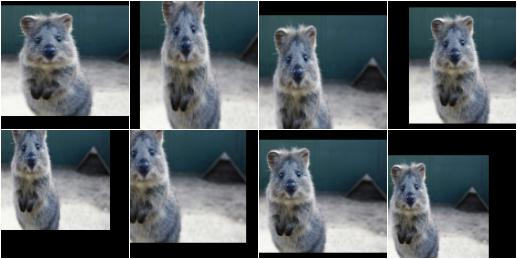In :
``````import torchvision.transforms as tt

stats = ((0.4914, 0.4822, 0.4465), (0.2023, 0.1994, 0.2010))
train_tfms = tt.Compose([tt.Resize((48,48)),
tt.RandomHorizontalFlip(p=.5),
tt.ToTensor(),
tt.Normalize(*stats,inplace=True)])
valid_tfms = tt.Compose([tt.ToPILImage(),
tt.ToTensor(),
tt.Resize((48,48)),
tt.Normalize(*stats)])
``````
In :
``````src="Dataset/Dataset/"
des="cropped/"``````
In :
``````from torchvision.datasets import ImageFolder

dataset = ImageFolder(root+des, train_tfms)
``````
In :
``````from random import choice

img,label=choice(dataset)
plt.imshow(img.permute(1,2,0).clamp(0,1))
print(dataset.classes[label].replace('_',' '))``````
```Bill Gates ```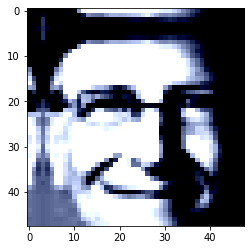In :
``````from torch.utils.data import random_split

train_len=int(len(dataset)*.9)
test_len=len(dataset)-train_len
train_ds, valid_ds=random_split(dataset,[train_len, test_len])
``````

Next, we can create data loaders using `DataLoader` class for retrieving images in batches. We'll use a relatively large batch size to utlize a larger portion of the GPU RAM. You can try reducing the batch size & restarting the kernel if you face an `out of memory` error.

In :
``````from torch.utils.data import DataLoader

batch_size = 200

train_dl = DataLoader(train_ds, batch_size, shuffle=True, num_workers=3, pin_memory=True)
valid_dl = DataLoader(valid_ds, batch_size*2, num_workers=3, pin_memory=True)``````

Let's take a look at some sample images from the training dataloader. To display the images, we'll need to denormalize the pixels values to bring them back into the range `(0,1)`.

In :
``````from torchvision.utils import make_grid

def denormalize(images, means, stds):
means = torch.tensor(means).reshape(1, 3, 1, 1)
stds = torch.tensor(stds).reshape(1, 3, 1, 1)
return images * stds + means

def show_batch(dl):
for images, labels in dl:
fig, ax = plt.subplots(figsize=(12, 12))
ax.set_xticks([]); ax.set_yticks([])
denorm_images = denormalize(images, *stats)
ax.imshow(make_grid(denorm_images[:64], nrow=8).permute(1, 2, 0).clamp(0,1))
break``````
In :
``show_batch(train_dl)``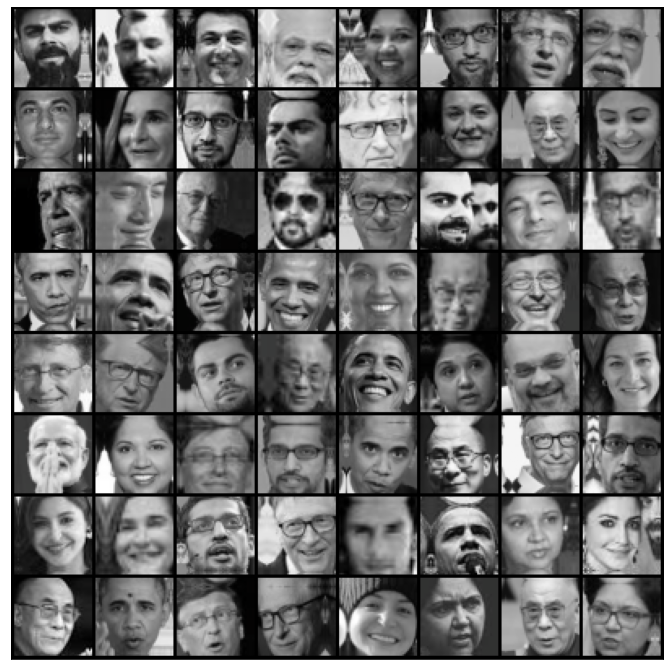### Using a GPU for faster training

As the sizes of our models and datasets increase, we need to use GPUs to train our models within a reasonable amount of time. GPUs contain hundreds of cores optimized for performing expensive matrix operations on floating-point numbers quickly, making them ideal for training deep neural networks. You can use GPUs for free on Google Colab and Kaggle or rent GPU-powered machines on services like Google Cloud Platform and Amazon Web Services.

You can use a Graphics Processing Unit (GPU) to train your models faster if your execution platform is connected to a GPU manufactured by NVIDIA. Follow these instructions to use a GPU on the platform of your choice:

• Google Colab: Use the menu option `Runtime` > `Change Runtime Type` and select `GPU` from the `Hardware Accelerator` dropdown.

• Kaggle: In the `Settings` section of the sidebar, select `GPU` from the `Accelerator` dropdown. Use the button on the top-right to open the sidebar.

• Binder: Notebooks running on Binder cannot use a GPU, as the machines powering Binder aren't connected to any GPUs.

• Linux: If your laptop/desktop has an NVIDIA GPU (graphics card), make sure you have installed the NVIDIA CUDA drivers.

• Windows: If your laptop/desktop has an NVIDIA GPU (graphics card), make sure you have installed the NVIDIA CUDA drivers.

• macOS: macOS is not compatible with NVIDIA GPUs.

We can check if a GPU is available and the required NVIDIA CUDA drivers are installed using `torch.cuda.is_available`.

In :
``torch.cuda.is_available()``
Out:
``True``

The following helper function is defined to ensure that our code uses the GPU if available and defaults to using the CPU if it isn't.

In :
``````def get_default_device():
"""Pick GPU if available, else CPU"""
if torch.cuda.is_available():
else:
In :
``````device = get_default_device()
device
``````
Out:
``device(type='cuda')``

Below helper function is used to move data and model to a chosen device.

In :
``````def to_device(data, device):
"""Move tensor(s) to chosen device"""
if isinstance(data, (list,tuple)):
return [to_device(x, device) for x in data]
return data.to(device, non_blocking=True)``````
In :
``````for images, labels in train_dl:
print(images.shape)
images = to_device(images, device)
print(images.device)
break``````
```torch.Size([200, 3, 48, 48]) cuda:0 ```

We also define a `DeviceDataLoader` class to wrap our existing data loaders and move batches of data to the selected device. Note that, we don't need to extend an existing class to create a PyTorch data loader. All we need is an `__iter__` method to retrieve batches of data and an `__len__` method to get the number of batches as shown.

In :
``````class DeviceDataLoader():
"""Wrap a dataloader to move data to a device"""
def __init__(self, dl, device):
self.dl = dl
self.device = device

def __iter__(self):
"""Yield a batch of data after moving it to device"""
for b in self.dl:
yield to_device(b, self.device)

def __len__(self):
"""Number of batches"""
return len(self.dl)``````

We can now wrap our data loaders using `DeviceDataLoader`.

In :
``````train_dl = DeviceDataLoader(train_dl, device)
``````

### Model with Residual Blocks and Batch Normalization

Our CNN model also has residual block, which adds the original input back to the output feature map obtained by passing the input through one or more convolutional layers as shown.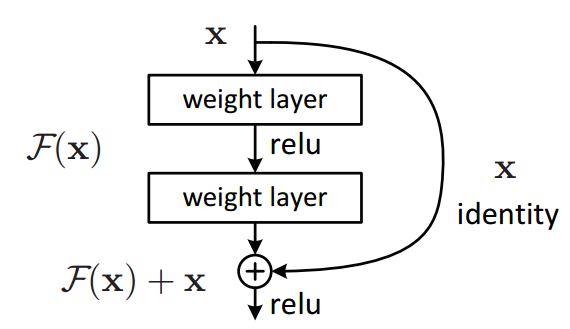This residual block produces a drastic improvement in the performance of the model. Also, after each convolutional layer, we'll add a batch normalization layer, which normalizes the outputs of the previous layer.

Reference:

We will be using the ResNet9 architecture as,In :
``````import torch.nn as nn
import torch.nn.functional as F

def accuracy(outputs, labels):
_, preds = torch.max(outputs, dim=1)

class ImageClassificationBase(nn.Module):
def training_step(self, batch):
images, labels = batch
out = self(images)                  # Generate predictions
loss = F.cross_entropy(out, labels) # Calculate loss
return loss

def validation_step(self, batch):
images, labels = batch
out = self(images)                    # Generate predictions
loss = F.cross_entropy(out, labels)   # Calculate loss
acc = accuracy(out, labels)           # Calculate accuracy
return {'val_loss': loss.detach(), 'val_acc': acc}

def validation_epoch_end(self, outputs):
batch_losses = [x['val_loss'] for x in outputs]
epoch_loss = torch.stack(batch_losses).mean()   # Combine losses
batch_accs = [x['val_acc'] for x in outputs]
epoch_acc = torch.stack(batch_accs).mean()      # Combine accuracies
return {'val_loss': epoch_loss.item(), 'val_acc': epoch_acc.item()}

def epoch_end(self, epoch, result):
print("Epoch [{}], last_lr: {:.5f}, train_loss: {:.4f}, val_loss: {:.4f}, val_acc: {:.4f}".format(
epoch, result['lrs'][-1], result['train_loss'], result['val_loss'], result['val_acc']))``````
In :
``````def conv_block(in_channels, out_channels, pool=False):
layers = [nn.Conv2d(in_channels, out_channels, kernel_size=3, padding=1),
nn.BatchNorm2d(out_channels),
nn.ReLU(inplace=True)]
if pool: layers.append(nn.MaxPool2d(2))
return nn.Sequential(*layers)

class ResNet9(ImageClassificationBase):
def __init__(self, in_channels, num_classes):
super().__init__()
# 3 X 48 X 48
self.conv1 = conv_block(in_channels, 64)  # 64 X 48 X 48
self.conv2 = conv_block(64, 128, pool=True) # 128 X 24 X 24
self.res1 = nn.Sequential(conv_block(128, 128),
conv_block(128, 128)) # 128 X 24 X 24

self.conv3 = conv_block(128, 256, pool=True)  # 256 X 12 X 12
self.conv4 = conv_block(256, 512, pool=True)  # 512 X 6 X 6
self.res2 = nn.Sequential(conv_block(512, 512),
conv_block(512, 512)) # 512 X 6 X 6

self.classifier = nn.Sequential(nn.MaxPool2d(6), # 512 X 1 X 1
nn.Flatten(),
nn.Dropout(0.2),
nn.Linear(512, num_classes))

def forward(self, xb):
out = self.conv1(xb)
out = self.conv2(out)
out = self.res1(out) + out
out = self.conv3(out)
out = self.conv4(out)
out = self.res2(out) + out
out = self.classifier(out)
return out``````
In :
``````model = to_device(ResNet9(3, 10), device)
model
``````
Out:
``````ResNet9(
(conv1): Sequential(
(0): Conv2d(3, 64, kernel_size=(3, 3), stride=(1, 1), padding=(1, 1))
(1): BatchNorm2d(64, eps=1e-05, momentum=0.1, affine=True, track_running_stats=True)
(2): ReLU(inplace=True)
)
(conv2): Sequential(
(0): Conv2d(64, 128, kernel_size=(3, 3), stride=(1, 1), padding=(1, 1))
(1): BatchNorm2d(128, eps=1e-05, momentum=0.1, affine=True, track_running_stats=True)
(2): ReLU(inplace=True)
(3): MaxPool2d(kernel_size=2, stride=2, padding=0, dilation=1, ceil_mode=False)
)
(res1): Sequential(
(0): Sequential(
(0): Conv2d(128, 128, kernel_size=(3, 3), stride=(1, 1), padding=(1, 1))
(1): BatchNorm2d(128, eps=1e-05, momentum=0.1, affine=True, track_running_stats=True)
(2): ReLU(inplace=True)
)
(1): Sequential(
(0): Conv2d(128, 128, kernel_size=(3, 3), stride=(1, 1), padding=(1, 1))
(1): BatchNorm2d(128, eps=1e-05, momentum=0.1, affine=True, track_running_stats=True)
(2): ReLU(inplace=True)
)
)
(conv3): Sequential(
(0): Conv2d(128, 256, kernel_size=(3, 3), stride=(1, 1), padding=(1, 1))
(1): BatchNorm2d(256, eps=1e-05, momentum=0.1, affine=True, track_running_stats=True)
(2): ReLU(inplace=True)
(3): MaxPool2d(kernel_size=2, stride=2, padding=0, dilation=1, ceil_mode=False)
)
(conv4): Sequential(
(0): Conv2d(256, 512, kernel_size=(3, 3), stride=(1, 1), padding=(1, 1))
(1): BatchNorm2d(512, eps=1e-05, momentum=0.1, affine=True, track_running_stats=True)
(2): ReLU(inplace=True)
(3): MaxPool2d(kernel_size=2, stride=2, padding=0, dilation=1, ceil_mode=False)
)
(res2): Sequential(
(0): Sequential(
(0): Conv2d(512, 512, kernel_size=(3, 3), stride=(1, 1), padding=(1, 1))
(1): BatchNorm2d(512, eps=1e-05, momentum=0.1, affine=True, track_running_stats=True)
(2): ReLU(inplace=True)
)
(1): Sequential(
(0): Conv2d(512, 512, kernel_size=(3, 3), stride=(1, 1), padding=(1, 1))
(1): BatchNorm2d(512, eps=1e-05, momentum=0.1, affine=True, track_running_stats=True)
(2): ReLU(inplace=True)
)
)
(classifier): Sequential(
(0): MaxPool2d(kernel_size=6, stride=6, padding=0, dilation=1, ceil_mode=False)
(1): Flatten(start_dim=1, end_dim=-1)
(2): Dropout(p=0.2, inplace=False)
(3): Linear(in_features=512, out_features=10, bias=True)
)
)``````

### Training the model

The following points are considered before we train the model. These are small but important improvements to our `fit` function.

• Learning rate scheduling: Instead of using a fixed learning rate, we will use a learning rate scheduler, which will change the learning rate after every batch of training. There are many strategies for varying the learning rate during training, and the one we'll use is called the One Cycle Learning Rate Policy, which involves starting with a low learning rate, gradually increasing it batch-by-batch to a high learning rate for about 30% of epochs, then gradually decreasing it to a very low value for the remaining epochs. Learn more...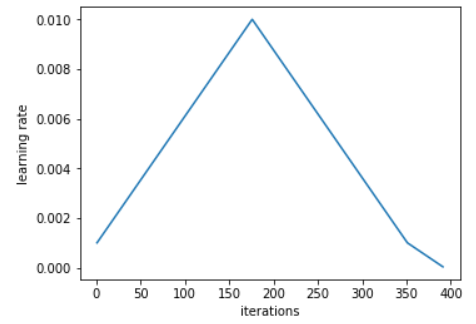• Weight decay: We also use weight decay, which is yet another regularization technique which prevents the weights from becoming too large by adding an additional term to the loss function. Learn more...

• Gradient clipping: Apart from the layer weights and outputs, it also helpful to limit the values of gradients to a small range to prevent undesirable changes in parameters due to large gradient values. This simple yet effective technique is called gradient clipping. Learn more...

We define `fit_one_cycle` function to incorporate these changes. We'll also record the learning rate used for each batch.

In :
``````@torch.no_grad()
model.eval()
outputs = [model.validation_step(batch) for batch in val_loader]
return model.validation_epoch_end(outputs)

def get_lr(optimizer):
for param_group in optimizer.param_groups:
return param_group['lr']

torch.cuda.empty_cache()
history = []

# Set up custom optimizer with weight decay
optimizer = opt_func(model.parameters(), max_lr, weight_decay=weight_decay)
# Set up one-cycle learning rate scheduler
sched = torch.optim.lr_scheduler.OneCycleLR(optimizer, max_lr, epochs=epochs,

for epoch in range(epochs):
# Training Phase
model.train()
train_losses = []
lrs = []
loss = model.training_step(batch)
train_losses.append(loss)
loss.backward()

optimizer.step()

# Record & update learning rate
lrs.append(get_lr(optimizer))
sched.step()

# Validation phase
result['train_loss'] = torch.stack(train_losses).mean().item()
result['lrs'] = lrs
model.epoch_end(epoch, result)
history.append(result)
return history``````

Let's see how the model performs on the validation set with the initial set of weights and biases.

In :
``````history = [evaluate(model, valid_dl)]
history
``````
Out:
``[{'val_acc': 0.1071428582072258, 'val_loss': 2.3058345317840576}]``

The initial accuracy is around 10%, as one might expect from a randomly initialized model (since it has a 1 in 10 chance of getting a label right by guessing randomly).

In :
``````epochs = 10
max_lr = 1e-3
weight_decay = 1e-4
``````
In :
``````%%time
history += fit_one_cycle(epochs, max_lr, model, train_dl, valid_dl,
weight_decay=weight_decay,
opt_func=opt_func)
``````
```Epoch , last_lr: 0.00026, train_loss: 2.6457, val_loss: 2.3249, val_acc: 0.1151 Epoch , last_lr: 0.00075, train_loss: 1.7251, val_loss: 1.9211, val_acc: 0.3968 Epoch , last_lr: 0.00100, train_loss: 1.2393, val_loss: 2.2545, val_acc: 0.3770 Epoch , last_lr: 0.00095, train_loss: 0.9410, val_loss: 0.8610, val_acc: 0.7698 Epoch , last_lr: 0.00081, train_loss: 0.7355, val_loss: 1.4765, val_acc: 0.5794 Epoch , last_lr: 0.00061, train_loss: 0.6034, val_loss: 0.5727, val_acc: 0.8294 Epoch , last_lr: 0.00039, train_loss: 0.5146, val_loss: 0.4886, val_acc: 0.8571 Epoch , last_lr: 0.00019, train_loss: 0.4535, val_loss: 0.4826, val_acc: 0.8611 Epoch , last_lr: 0.00005, train_loss: 0.3949, val_loss: 0.4198, val_acc: 0.8968 Epoch , last_lr: 0.00000, train_loss: 0.3898, val_loss: 0.3690, val_acc: 0.9008 CPU times: user 4.36 s, sys: 3.12 s, total: 7.47 s Wall time: 39.2 s ```

Our model trained to over 90% accuracy in less than 1 minute!

Let's plot the valdation set accuracies to study how the model improves over time.

In :
``````def plot_accuracies(history):
accuracies = [x['val_acc'] for x in history]
plt.plot(accuracies, '-x')
plt.xlabel('epoch')
plt.ylabel('accuracy')
plt.title('Accuracy vs. No. of epochs');``````
In :
``plot_accuracies(history)``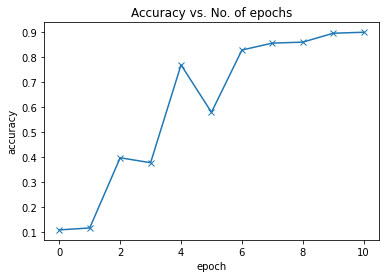We can also plot the training and validation losses to study the trend.

In :
``````def plot_losses(history):
train_losses = [x.get('train_loss') for x in history]
val_losses = [x['val_loss'] for x in history]
plt.plot(train_losses, '-bx')
plt.plot(val_losses, '-rx')
plt.xlabel('epoch')
plt.ylabel('loss')
plt.legend(['Training', 'Validation'])
plt.title('Loss vs. No. of epochs');``````
In :
``plot_losses(history)``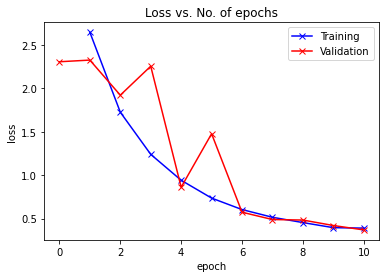It can be noted from the trend that our model isn't overfitting to the training data just yet.

Finally, let's visualize how the learning rate changed over time, batch-by-batch over all the epochs.

In :
``````def plot_lrs(history):
lrs = np.concatenate([x.get('lrs', []) for x in history])
plt.plot(lrs)
plt.xlabel('Batch no.')
plt.ylabel('Learning rate')
plt.title('Learning Rate vs. Batch no.');``````
In :
``plot_lrs(history)``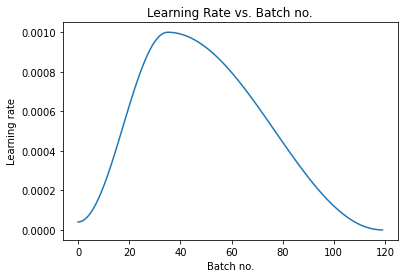The learning rate starts at a low value, and gradually increases for 30% of the iterations to a maximum value, and then gradually decreases to a very small value.

### Testing the model

While we have been tracking the overall accuracy of a model so far, it's also a good idea to look at model's results on some sample images. Let's test out our model with some images from the dataset.

Let's define a helper function `predict_image`, which returns the predicted label for a single image tensor.

In :
``````def predict_image(img, model):
# Convert to a batch of 1
xb = to_device(img.unsqueeze(0), device)
# Get predictions from model
yb = model(xb)
# Pick index with highest probability
_, preds  = torch.max(yb, dim=1)
# Retrieve the class label
return dataset.classes[preds.item()]``````

Let's predict image from validation dataset

In :
``````img, label=choice(valid_ds)
plt.imshow(img.permute(1,2,0).clamp(0,1))
plt.imshow(denormalize(img,*stats).squeeze(0).permute(1,2,0).clamp(0,1))
predict_image(img, model), dataset.classes[label]
``````
Out:
``('Sundar_Pichai', 'Sundar_Pichai')``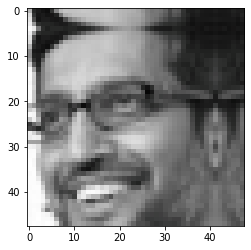Let us also predict image from raw dataset

In :
``````person=choice(os.listdir(root+src)) # random person
img=choice(os.listdir(root+src+person)) # random person's image
path=os.path.join(root,src,person,img)
crop=get_face(path)
if crop is not None:
```Bill_Gates ```